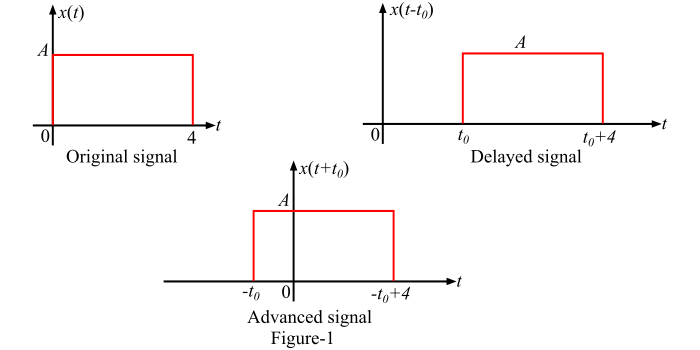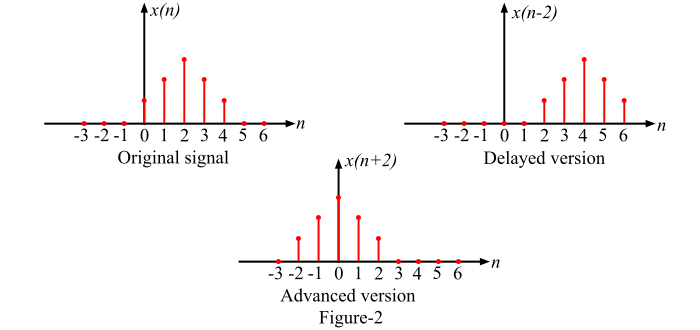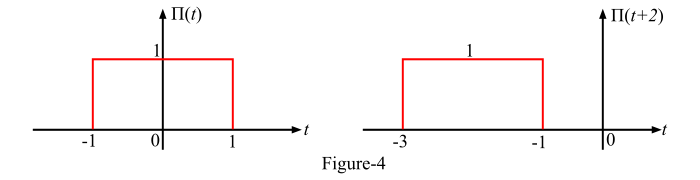# What is the Time Shifting Operation on Signals?

## What is Time Shifting?

Time shifting or Shifting of a signal in time means that the signal may be either delayed in the time axis or advanced in the time axis.

## Time Shifting of Continuous-Time Signal

The time shifting of a continuous time signal x(t) is represented as,

𝑦(𝑡) = 𝑥(𝑡 − 𝑡0)

The time-shifting of a signal results in the time delay or time advancement. The above expression shows that the signal y(t) can be obtained by time shifting the signal x(t) by t0 units. If t0 is positive in the above expression, then the shift of the signal is to the right and hence the time shifting delays the signal. On the other hand, if t0 is negative, then the shift of the signal is to the left and hence the shifting advances the signal in the time axis.

The time shifting of a continuous-time signal x(t) is shown in Figure-1.## Time Shifting of a Discrete-Time Signal

The time shifting operation of a discrete time signal x(n) is represented as

𝑦(𝑛) = 𝑥(𝑛 − 𝑛0)

This equation shows that the signal y(n) can be obtained by time-shifting the signal x(n) by 𝑛0 units. If the value of 𝑛0 is positive, then the shift of the signal is to the right and the resulting signal is the delayed version. Whereas, if the value of 𝑛0 is negative, then the shift of the signal is to the left and it is the time advanced version of the signal.Figure-2 shows an arbitrary discrete-time signal x(n). Here, the signal x(n – 2) is shown which is obtained by shifting of the signal x(n) to the right by 2 units, i.e., the signal x(n) is delayed by 2 units. Also, the signal x(n + 2) is obtained by shifting x(n) to the left by 2 units, i.e., the signal x(n) is advanced by 2 units.

## Numerical Example

Sketch the following signals −

• (𝑡) = 3𝑟(𝑡 − 3)
• (𝑡) = Π(𝑡 + 2)

### Solution

• Given signal is,

𝑥(𝑡) = 3𝑟(𝑡 − 3)

The signal [3𝑟(𝑡 − 3)] can be obtained by first drawing the ramp signal [3r(t)] with slope of 3 as shown in Figure-3. Then, the shifting of the signal to the right by 3 units to obtain the given signal as shown in Figure-3.• Given signal is,

𝑥(𝑡) = Π(𝑡 + 2)

The given signal [Π(𝑡 + 2)] can be obtained by first drawing Π(𝑡) as shown in Figure-4 and then shifting it to the left by 2 units to obtained the given signal as shown in Figure-4.